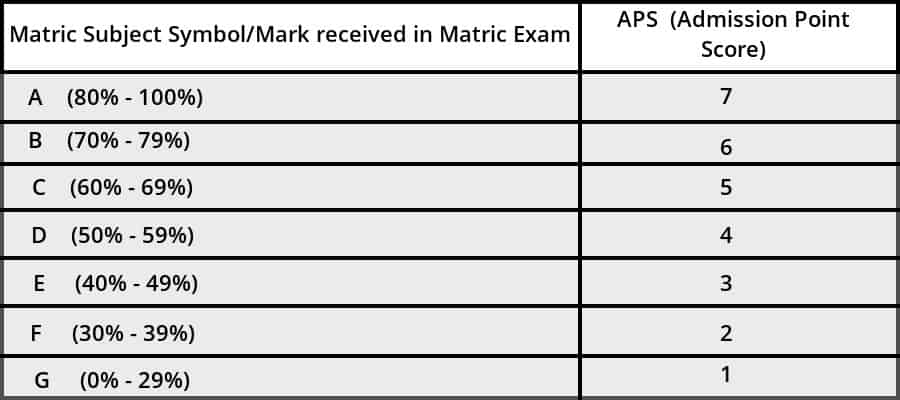# What is my APS for University Admission?

“What is my APS?” is a question that most Matric pupils and first time students  want to know the answer to when applying to study at a university.

## What does APS stand for?

APS stands for Admission Point Score. This is the score that universities use to see if you qualify for a specific course. You can work out your APS using your final Matric results.

You need to get your APS (Admission Point Score) mark before you can apply for a course at a university. If you want to study further after matric, your APS will be used to calculate whether or not you qualify to study a certain course at an institution.

## How do I get my APS?

To calculate your APS, you have to have your final Matric examination certificate. Your top 7 Matric subject’s marks are changed into a point system that works out your APS (see diagram 1 below).

### APS CalculatorUnderstanding the table:

• You get a point for each Matric subject that you wrote in the final examination.
• The number of points you get for each of your final Matric examination subjects will depend on what percentage you get for each subject.
• The points get for each subject should then be added up. This total will be your APS.
• (See diagram 2 below as an example of how you calculate your APS).

### APS Calculator Example(The total points in the above diagram is 40 points)

## How do universities use the APS to allow you to apply for a course at the university?

• Universities use your total APS point to see if you qualify to study for the specific course at that you are applying for.
• Take note: Each university allocates the amount of APS points needed to study a course differently. This means that a course at one university won’t need the same APS points as the same course at another university.
• It is very important that you make absolutely sure you find out what a university’s specified minimum APS points are for a specific course before you apply, as well as all the other specifications.
• For example: You want to apply for a teaching course at a university. One university might state that your minimum APS must be 30. However, another university might state that the minimum APS needed to apply for a teaching course is 20.
• Also important to remember:  Make sure that you always study hard to get the highest marks/grades you are capable of getting. This is because it is not always enough to have only the bare minimum necessary to get accepted for the degree you want to study.

## In short:

• You need a good APS to be accepted at the university of your choice.